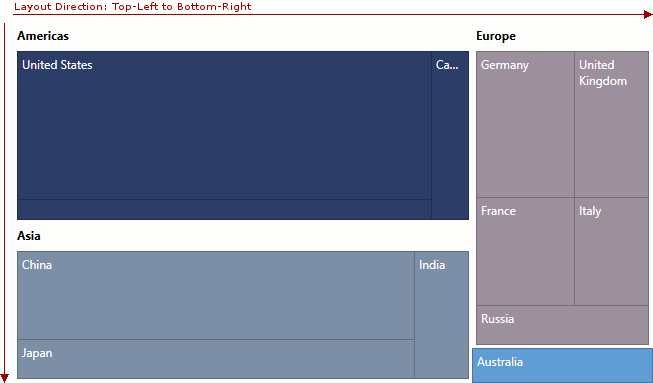# Layout Algorithms

This topic describes the layout algorithms used by the TreeMap control. It consists of the following sections.

## Overview

The TreeMap control allows you to specify the algorithm used to layout tree map items. The Squarified algorithm is used by default.

To change the algorithm used to arrange tree map items, assign the required TreeMapLayoutAlgorithmBase class descendant object to the TreeMapControl.LayoutAlgorithm property.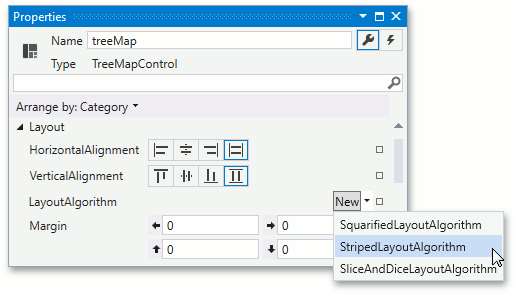After that, customize algorithm settings. For example, all predefined algorithms support fill direction customization. To do this, use the TreeMapLayoutAlgorithm.Direction property.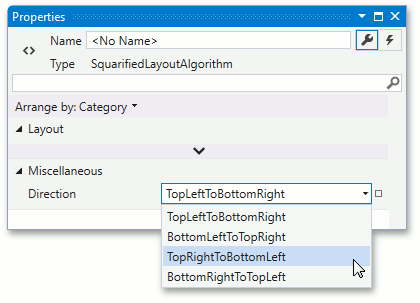The next sections describe predefined algorithm behavior.

## Slice and Dice Layout Algorithm

This layout algorithm divides the space between items, slicing it in the specified direction depending on item value.

The following image demonstrates the country GDP tree map with a layout calculated using this algorithm.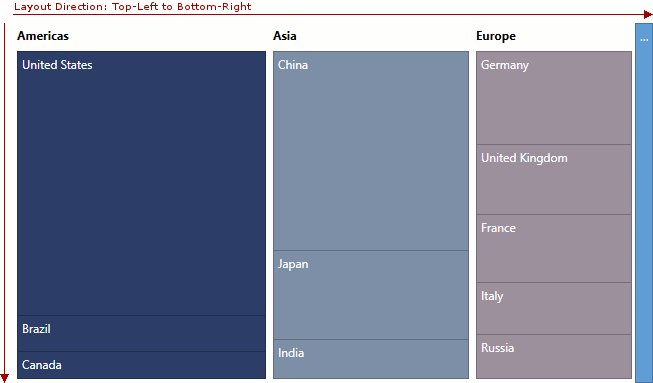## Squarified Layout Algorithm

The Squarified algorithm layouts items so that width/height ratio will be closer to 1. In other words, this algorithm tries to make items similar to square as far as it possible.

The following image demonstrates the tree map layout calculated using this algorithm.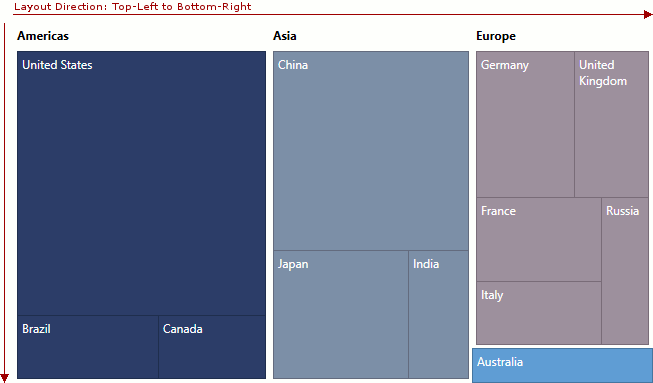## Striped Layout Algorithm

This algorithm is a modified version of the Squarified algorithm. The main difference is that for all items width/height ratio is close. E.g. algorithm tries to make all item widths larger than heights.

The following image demonstrates the layout calculated using this algorithm.